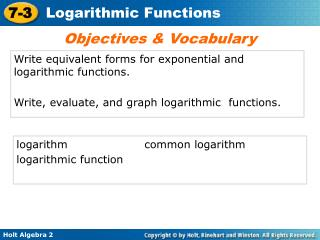DownloadDownload PresentationWrite equivalent forms for exponential and logarithmic functions.

# Write equivalent forms for exponential and logarithmic functions.

Télécharger la présentation## Write equivalent forms for exponential and logarithmic functions.

- - - - - - - - - - - - - - - - - - - - - - - - - - - E N D - - - - - - - - - - - - - - - - - - - - - - - - - - -
##### Presentation Transcript

1. Objectives & Vocabulary Write equivalent forms for exponential and logarithmic functions. Write, evaluate, and graph logarithmic functions. logarithm common logarithm logarithmic function

2. 2 1 27 3 B. Change log279 = to exponential form. Notes #1-2 Rewrite in other form a. b. c. 3A. Change 64 = 1296 to logarithmic form Calculate (without a calculator). 4. log864 5. log3

3. Reading Math Read logba=x, as “the log base b of a is x.” Notice that the log is the exponent. You can write an exponential equation as a logarithmic equation and vice versa.

4. 1 1 3 3 8 = 2 1 4–2 = 16 Example 1: Converting from Logarithmic to Exponential Form Write each logarithmic form in exponential equation. The base of the logarithm becomes the base of the power. 91 = 9 The logarithm is the exponent. 29 = 512 A logarithm can be a negative number. 1 16 Any nonzero base to the zero power is 1. b0 = 1

5. 1 1 log6 = –1 6 6 1 1 2 log255 = 2 Example 2: Converting from Exponential to Logarithmic Form Write each exponential equation in logarithmic form. The base of the exponent becomes the base of the logarithm. log3243 = 5 The exponent is the logarithm. log1010,000 = 4 An exponent (or log) can be negative. logac =b The log (and the exponent) can be a variable.

6. Notes #1 Write each exponential equation in logarithmic form. The base of the exponent becomes the base of the logarithm. log981 = 2 a. log327 = 3 b. The exponent of the logarithm. The log (and the exponent) can be a variable. logx1 = 0 c.

7. 1 1 2 2 –3 = 8 Notes #2 Write each logarithmic form in exponential equation. The base of the logarithm becomes the base of the power. 103 = 1000 122 = 144 The logarithm is the exponent. An logarithm can be negative.

8. A logarithm is an exponent, so the rules for exponents also apply to logarithms. You may have noticed the following properties in the last example.

9. A logarithm with base 10 is called a common logarithm. If no base is written for a logarithm, the base is assumed to be 10. For example, log 5 = log105. You can use mental math to evaluate some logarithms.

10. Example 3A: Evaluating Logarithms by Using Mental Math Evaluate by without a calculator. log 0.01 10? = 0.01 The log is the exponent. 10–2= 0.01 Think: What power of 10 is 0.01? log 0.01 = –2

11. Example 3B: Evaluating Logarithms by Using Mental Math Evaluate without a calculator. log5 125 5? = 125 The log is the exponent. log5125 = 3

12. 1 5 1 log5 = –1 5 Example 3C/3D: Evaluating Logarithms by Using Mental Math Evaluate without a calculator. 3c. log5 3d. log250.04 log250.04 = –1

13. Because logarithms are the inverses of exponents, the inverse of an exponential function, such as y = 2x, is a logarithmic function, such as y = log2x. You may notice that the domain and range of each function are switched. The domain of y = 2x is all real numbers (R), and the range is {y|y > 0}. The domain of y = log2x is {x|x > 0}, and the range is all real numbers (R).

14. f(x) = x Graph f(x) = x by using a table of values. 1 1 1 2 4 2 1 1 2 2 Example 4A: Graphing Logarithmic Functions Use the x-values {–2, –1, 0, 1, 2}. Graph the function and its inverse. Describe the domain and range of the inverse function.

15. 1 1 2 2 1 1 2 4 Example 4A Continued To graph the inverse, f–1(x) = log x,by using a table of values. The domain of f–1(x) is {x|x > 0}, and the range is R.

16. 2 1 3 27 B. Change log279 = to exponential form. 2 27 = 9 3 Notes (continued) 3A. Change 64 = 1296 to logarithmic form log61296= 4 Calculate the following using mental math (without a calculator). 4. log864 2 5. log3 –3

17. 6. Use the x-values {–1, 0, 1, 2} to graph f(x) = 3x Then graph its inverse. Describe the domain and range of the inverse function. Notes (graphing) D: {x > 0}; R: all real numbers